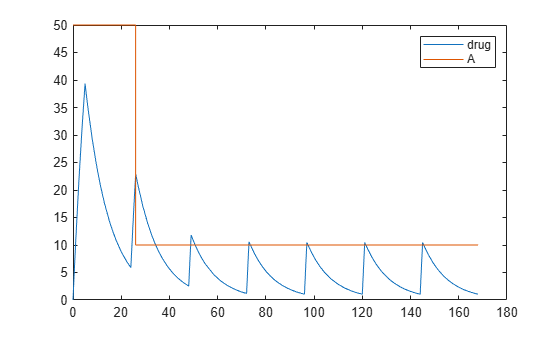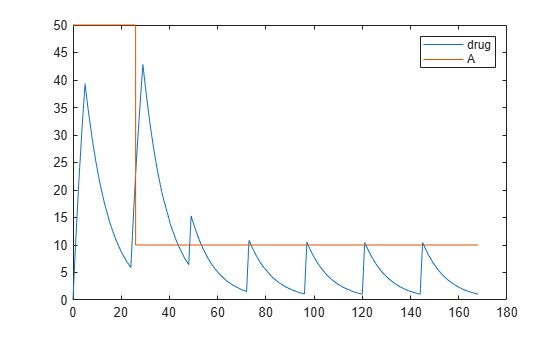# EventMode

Determine how events that change dose parameters affect in-progress dosing

## Description

`EventMode` is a property of `RepeatDose` and `ScheduleDose` objects. This property determines whether to continue the ongoing dose, that is, a dose with a nondefault infusion rate or dose duration, to completion when an event changes a parameter that is referenced by a dose property. Dose properties that you can parameterize are: `Amount`, `Rate`, `Interval`, `StartTime`, `RepeatCount`, `LagParameterName`, and `DurationParameterName`.

If `EventMode` is set to `'continue'`, the ongoing dose continues to completion when the event changes dose parameters, and updated parameters affect only subsequent doses. If `EventMode` is set to `'stop'`, the ongoing dose stops immediately when dose parameters change, and subsequent doses use the updated parameters.

To decide whether a parameter has been changed, SimBiology compares the old value of a parameter to the new value. For instance, the following event does not change the `doseStartTime` parameter value: ```addevent(model,'time >= 5','doseStartTime = doseStartTime * 1')```.

Any change in dose parameters affects the schedule of doses generated. If the simulation reaches a time point for a scheduled dose, the dose is applied. If an event changes dose parameters, SimBiology updates the dose schedule, ignores any doses scheduled before the current simulation time, and applies only the subsequent doses. Suppose that you have parameterized the `StartTime` property of a dose. Updating the parameter with an event causes to regenerate the dose schedule. If there are any previously-scheduled doses before the current simulation time, they are ignored.

By default, SimBiology® uses the following MATLAB® expression to generate a list of dose times (dose schedule) whenever an event changes any dose parameter, using the updated parameter values:

,

where StartTime, RepeatCount, and Interval are properties of the dose object. Lag is the time lag parameter for a dose, referenced by the `LagParameterName` property.

## Characteristics

 Applies to Objects: `RepeatDose`, `ScheduleDose` Data type Character vector Data values `'stop'` (default) or `'continue'` Access Read/write

## Examples

expand all

Create a simple model with linear elimination, an amount parameter, and a rate parameter.

```model = sbiomodel('simple model'); compartment = addcompartment(model,'Central',1); compartment.CapacityUnits = 'liter'; species = addspecies(model,'drug'); species.InitialAmountUnits = 'milligram'; % Elimination rate elimParam = addparameter(model,'kel',0.1); elimParam.ValueUnits = '1/hour'; % Elimination reaction reaction = addreaction(model,'drug -> null'); reaction.ReactionRate = 'kel*drug'; % Add amount and rate parameters amountParam = addparameter(model,'A',50); amountParam.ConstantValue = false; amountParam.ValueUnits = 'milligram'```
```amountParam = SimBiology Parameter Array Index: Name: Value: Units: 1 A 50 milligram ```
```rateParam = addparameter(model,'R',10); rateParam.ValueUnits = 'milligram/hour'```
```rateParam = SimBiology Parameter Array Index: Name: Value: Units: 1 R 10 milligram/hour ```

Create a dose with its `Amount` and `Rate` properties set to the amount and rate parameters 'A' and 'R', respectively.

```dose = adddose(model,'adaptive dose','repeat'); dose.Amount = 'A'; dose.Rate = 'R';```

Set other dose properties.

```dose.TargetName = 'drug'; dose.StartTime = 0; dose.TimeUnits = 'hour'; dose.Interval = 24; dose.RepeatCount = 7;```

Prepare the configuration set to simulate the model for 7 days.

```configset = getconfigset(model); configset.StopTime = 7*24; configset.TimeUnits = 'hour';```

Add an event to reset the dose amount to 10 at time >= 26.

`event = addevent(model,'time >= 26','A = 10');`

Set the `EventMode` property to 'stop'. This setting causes any ongoing dose event to stop at 26 hours.

`dose.EventMode = 'stop';`

Simulate the model. The second dose event stops at 26 hours, and the subsequent dose events continue with the new dose amount of 10.

```[time, drugAndAmount] = sbiosimulate(model,dose); figure plot(time, drugAndAmount); legend('drug','A');```Alternatively, you can allow the ongoing dose event to finish before applying the new dose amount by setting `EventMode` to 'continue'.

`dose.EventMode = 'continue';`

Simulate the model. In this case, the second dose event continues to 26 hours.

```[time, drugAndAmount] = sbiosimulate(model,dose); figure plot(time, drugAndAmount); legend('drug','A');```